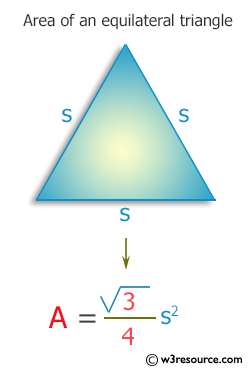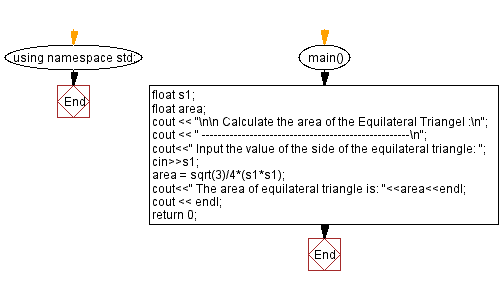﻿ C++ Exercises: Calculate area of an equilateral triangle - w3resource

# C++ Exercises: Calculate area of an equilateral triangle

## C++ Basic: Exercise-53 with Solution

Write a C++ program to calculate the area of an equilateral triangle.

Pictorial Presentation:Sample Solution:

C++ Code :

``````#include<iostream>
using namespace std;
#include<math.h>

main(){

float s1;
float area;
cout << "\n\n Calculate the area of the Equilateral Triangle :\n";
cout << " ----------------------------------------------------\n";

cout<<" Input the value of the side of the equilateral triangle: ";
cin>>s1;
area = sqrt(3)/4*(s1*s1);
cout<<" The area of equilateral triangle is: "<<area<<endl;
cout << endl;
return 0;
}
``````

Sample Output:

```Calculate the area of the Equilateral Triangle :
----------------------------------------------------
Input the value of the side of the equilateral triangle: 5
The area of equilateral triangle is: 10.8253
```

Flowchart:C++ Code Editor:

What is the difficulty level of this exercise?

﻿

## C++ Programming: Tips of the Day

Why is there no std::stou?

The most pat answer would be that the C library has no corresponding "strtou", and the C++11 string functions are all just thinly veiled wrappers around the C library functions: The std::sto* functions mirror strto*, and the std::to_string functions use sprintf.

Ref: https://bit.ly/3wtz2qA

We are closing our Disqus commenting system for some maintenanace issues. You may write to us at reach[at]yahoo[dot]com or visit us at Facebook